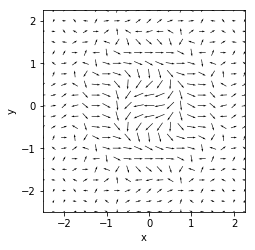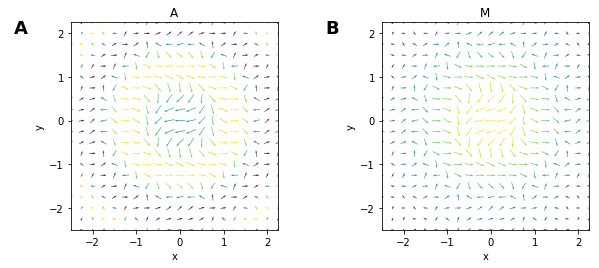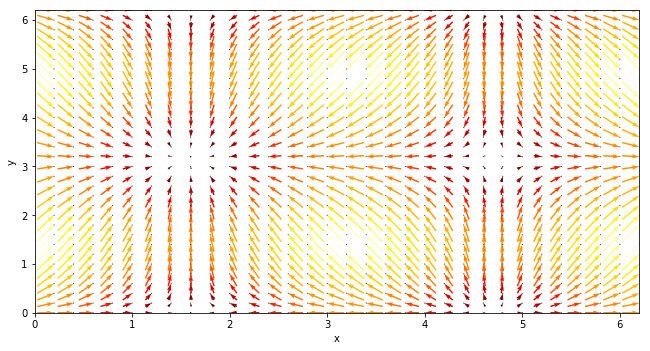# Vectorfield¶

Title
VectorField Element
Dependencies
Matplotlib
Backends
Matplotlib
Bokeh
In :
import numpy as np
import holoviews as hv
from holoviews import dim, opts

hv.extension('matplotlib')A VectorField plot displays velocity vectors as arrows at x and y positions with angle and magnitude components (or alternatively U, V components). The element accepts the usual columnar format passing the (x, y, angles, magnitudes) components as a tuple:

In :
x,y  = np.mgrid[-10:10,-10:10] * 0.25
sine_rings  = np.sin(x**2+y**2)*np.pi+np.pi
exp_falloff = 1/np.exp((x**2+y**2)/8)

vector_data = (x, y, sine_rings, exp_falloff)
hv.VectorField(vector_data).opts(magnitude='Magnitude')

Out:As you can see above, here the x and y positions are chosen to make a regular grid and the angles supplied to a VectorField are expressed in radians. The arrow angles follow a sinsoidal ring pattern, and the arrow lengths fall off exponentially from the center, so this plot has four dimensions of data (direction and length for each x,y position).

Using the .opts method, we can also use color as a redundant indicator to the direction or magnitude:

In :
anglecolor = hv.VectorField(vector_data).opts(
opts.VectorField(title='A', magnitude='Magnitude', color='Angle'))
magcolor = hv.VectorField(vector_data).opts(
opts.VectorField(title='M', magnitude='Magnitude', color='Magnitude'))
anglecolor + magcolor

Out:By default the magnitudes are rescaled to the minimum distance between individual arrows, to disable this rescaling set rescale_lengths=False use a dimension value transform to scale the size of the arrows, e.g. below we normalize the 'Magnitude' dimension values and then scale them by a factor of 0.2. This allows fixed scaling even when plotting arrows in an animation. Here we will vary the arrow angle with a Phase dimension and also add this angle to the magnitude data, showing the arrow angles and magnitudes varying. Due to the fixed scaling we can make out the differences across frames:

In :
hmap = hv.HoloMap({phase: hv.VectorField((x, y,(vector_data+phase)%np.pi*2, vector_data+np.abs(phase)))
for phase in np.linspace(-np.pi,np.pi,5)}, kdims='Phase')

hmap.opts(opts.VectorField(color='Angle', magnitude=dim('Magnitude').norm()*0.2, rescale_lengths=False))

Out:

Vectors are also often expressed through U and V components we can easily transform these to a magnitude and angle:

In :
xs, ys = np.arange(0, 2 * np.pi, .2), np.arange(0, 2 * np.pi, .2)
X, Y = np.meshgrid(xs, ys)
U = np.cos(X)
V = np.sin(Y)

# Convert U, V to magnitude and angle
mag = np.sqrt(U**2 + V**2)
angle = (np.pi/2.) - np.arctan2(U/mag, V/mag)


VectorField also allows defining the pivot point of the vectors. We can for instance define pivot='tip' to pivot around the tip of the arrow. To make this clearer we will mark the pivot points:

In :
vectors = hv.VectorField((xs, ys, angle, mag))
points = hv.Points((X.flat, Y.flat))

(vectors * points).opts(
opts.Points(color='black', s=1),
opts.VectorField(aspect=2, color='Magnitude', cmap='fire', fig_size=300, magnitude='Magnitude', pivot='tip', scale=0.8))

Out:For full documentation and the available style and plot options, use hv.help(hv.VectorField).